# Lathe

95% of the components manufactured on the lathe comply with the standard, of which 80% of the components are first-class. How likely can we expect a manufactured part to be first class?

Result

p =  76 %

#### Solution:Leave us a comment of example and its solution (i.e. if it is still somewhat unclear...):Be the first to comment!#### To solve this example are needed these knowledge from mathematics:

Our percentage calculator will help you quickly calculate various typical tasks with percentages. Would you like to compute count of combinations?

## Next similar examples:

1. Fruit tea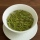Tea contains 7% of fruit components and 12% of sugar in this component. How many percents of sugar is represented in the whole tea?
2. CandiesIn the box are 12 candies that look the same. Three of them are filled with nougat, five by nuts, four by cream. At least how many candies must Ivan choose to satisfy itself that the selection of two with the same filling? ?
3. Mixed with percentagesCalculate 33 1/3% of 570.
4. Probability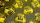What are the chances that the lottery, in which the numbers are drawn 5 of 50 you win the first prize?
5. Win in raffle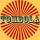The raffle tickets were sold 200, 5 of which were winning. What is the probability that Peter, who bought one ticket will win?
6. Probability - tickets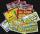What is the probability that when you have 25 tickets in 5000 that you wins the first (one) prize?
7. First manWhat is the likelihood of a random event where are five men and seven women first will leave the man?
8. Today in schoolThere are 9 girls and 11 boys in the class today. What is the probability that Suzan will go to the board today?
9. Sum 10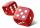What is the probability that two dice throw twice in a row will result the sum of 10?
10. BallsThe urn is 8 white and 6 black balls. We pull 4 randomly balls. What is the probability that among them will be two white?
11. Probabilities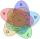If probabilities of A, B and A ∩ B are P (A) = 0.62 P (B) = 0.78 and P (A ∩ B) = 0.26 calculate the following probability (of union. intersect and opposite and its combinations):55%+36%+88%+71%+100=63% what is whole (X)? Percents can be added directly together if they are taken from the same whole, which means they have the same base amount. .. . You would add the two percentages to find the total amount.We want to prove the sentence: If the natural number n is divisible by six, then n is divisible by three. From what assumption we started?If 5% more is gained by selling an article for Rs. 350 than by selling it for Rs. 340, the cost of the article is: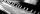If Suzan practicing 10 minutes at Monday; every other day she wants to practice 2 times as much as the previous day, how many hours and minutes will have to practice on Friday?If two consecutive times we increase the price of the product by 20%, how many % is higher final price than the original?I borrow 25,000 to 6.9% p.a.. I pay 500 per month. How much will I pay and for how long?